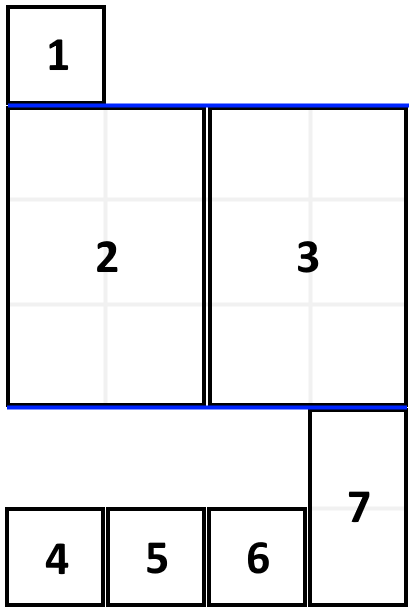## Problem

Problem ID: 1196

Title: Filling Bookcase Shelves

Difficulty: Medium

Description:

You are given an array books where books[i] = [thicknessi, heighti] indicates the thickness and height of the ith book. You are also given an integer shelfWidth.

We want to place these books in order onto bookcase shelves that have a total width shelfWidth.

We choose some of the books to place on this shelf such that the sum of their thickness is less than or equal to shelfWidth, then build another level of the shelf of the bookcase so that the total height of the bookcase has increased by the maximum height of the books we just put down. We repeat this process until there are no more books to place.

Note that at each step of the above process, the order of the books we place is the same order as the given sequence of books.

• For example, if we have an ordered list of 5 books, we might place the first and second book onto the first shelf, the third book on the second shelf, and the fourth and fifth book on the last shelf.

Return the minimum possible height that the total bookshelf can be after placing shelves in this manner.

Example 1:Input: books = [[1,1],[2,3],[2,3],[1,1],[1,1],[1,1],[1,2]], shelf_width = 4
Output: 6
Explanation:
The sum of the heights of the 3 shelves is 1 + 3 + 2 = 6.
Notice that book number 2 does not have to be on the first shelf.


Example 2:

Input: books = [[1,3],[2,4],[3,2]], shelfWidth = 6
Output: 4


Constraints:

• 1 <= books.length <= 1000
• 1 <= thicknessi <= shelfWidth <= 1000
• 1 <= heighti <= 1000

None

## Solution

General Idea

Starting from the last book, we find the current minimum possible height with the current book at the start of the row.

### Implementation

DP State:

• dp[i]: represents the minimum height of books [i,...,n-1] with book i at the start of the row

DP Transition:

• For all j >= i and sum of books[i] + ... books[j] <= shelfWidth, dp[i] = min( dp[i], max(books[i], ..., books[j]) + dp[j+1])
• Represents trying to add as many books to the row with book i at the start of the row. For each new book added to the row you can find the minimum height by adding the minimum of the books in the current row and the minimum height of the shelf with the next book at a new row.
class Solution {
public:
int minHeightShelves(vector<vector<int>>& books, int shelfWidth) {
int n = books.size();
vector<int> dp(n, 1e9);
for (int i = n-1; i >= 0; i--) {
int w = 0;
int h = 0;
for (int j = i ; j < n; j++) {
w += books[j];
h = max(h, books[j]);
if (w > shelfWidth) break;